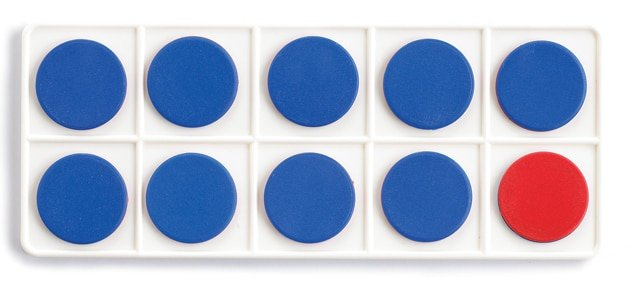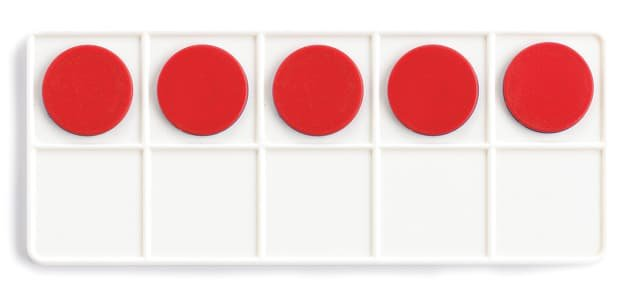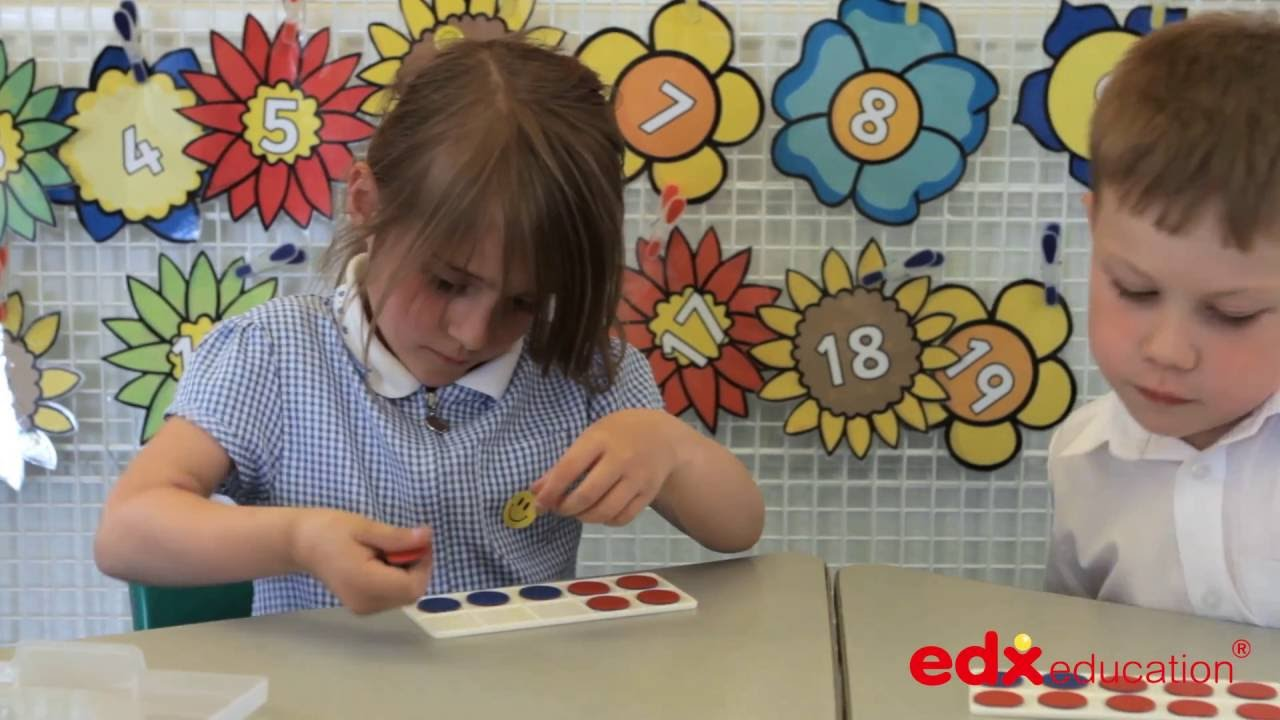# Ten Frames

#### • Number: Counting, Operations

The ten frame is a simple but effective mathematics manipulative, especially when paired up with two-sided, two-colour counters. The two colours help to focus students on the mathematics.9 + 6
becomes10 + 5

### Tens Frames Videos### Mathematical Language

Add, less, more, subtract, take away.

### Using ten Frames

Note that ten frames are typically filled in two different ways. One method involves filling the top row from left to right first and then the second row. This technique anchors on the five in the top row. This is similar to the Rekenrek.

An alternative way to fill the ten frame is in a pair-wise configuration. This method helps to
emphasise odd and evenness. Notice how 7 looks using this filling method.

Students can ‘see’ that it would take another 3 counters to complete the ten frame.

Ten Frames may be used to support students with addition and subtraction.

Students can learn the facts that add to ten by placing counters of one colour onto the ten frame and then either counting the remaining cells or placing counters of a different colour to calculate how many more to ten.

### Typical Classroom Requirements

#### A class set:

– Students would need at least one ten frame and ten two-colour two-sided counters each.
Often they will need two ten frames, but can work in pairs.

### Support and Complementary Materials

To effectively use ten frames requires the use of two-colour two-sided counters. There are several games based on the use of ten frames such as Race to Twenty.